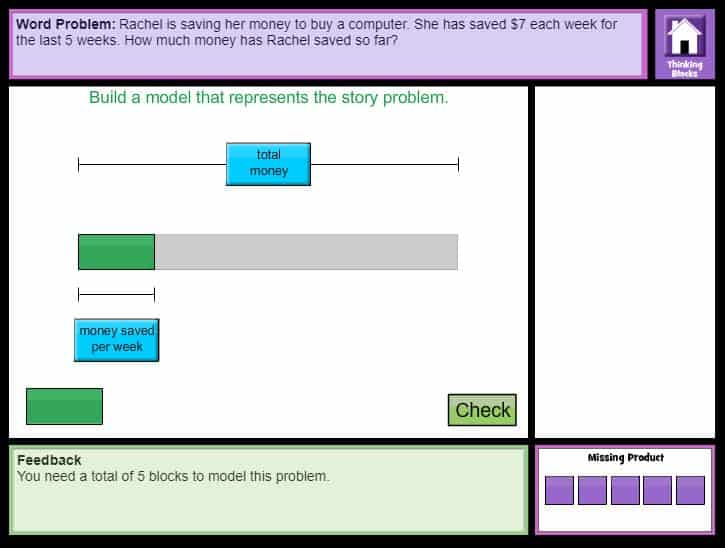## Interactive Problem Solving - Multiplication and Division

Thinking Blocks Interactive Tutorials– Model and solve word problems with multiplication and division.  Online interactive tutorial (Third-Fifth).

#### Online Word Problems with Multiplication and Division Level 2CCSS.MATH.CONTENT.3.OA.A.3
Use multiplication and division within 100 to solve word problems in situations involving equal groups, arrays, and measurement quantities, e.g., by using drawings and equations with a symbol for the unknown number to represent the problem.

CCSS.MATH.CONTENT.4.OA.A.2
Multiply or divide to solve word problems involving multiplicative comparison, e.g., by using drawings and equations with a symbol for the unknown number to represent the problem, distinguishing multiplicative comparison from additive comparison.